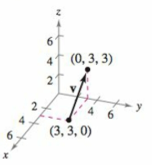Chapter 11.2, Problem 47E

Chapter
Section
Textbook Problem

# Finding the Component Form of a Vector in Space In Exercises 45-48, (a) find the component form of the vector v, (b) write the vector using standard unit vector notation, and (c) Sketch the vector with its initial point at the origin.(a)

To determine

To calculate: The component form of the vector v with the help of the graph provided in the question.

Explanation

Given:

Refer to the graph provided in the question.

Formula used:

The component form for any vector x directed by a line segment with end points P=(p1,p2,p3) and Q=(q1,q2,q3) is:

x=v1,v2,v3=q1p1,q2p2,q3p3

Calculation:

Refer to the graph provided in question to find out the endpoints, that are: (0,3,3),(3,3,0)

(b)

To determine

To calculate: The standard unit notation of the vector v=3,0,3.

(c)

To determine

To graph: The vector v=3i+3k with initial point at (0,0,0).

### Still sussing out bartleby?

Check out a sample textbook solution.

See a sample solution

#### The Solution to Your Study Problems

Bartleby provides explanations to thousands of textbook problems written by our experts, many with advanced degrees!

Get Started

#### Find a formula for the inverse of the function. 26. y=1ex1+ex

Single Variable Calculus: Early Transcendentals, Volume I

#### In Exercises 19-24, find the functions f + g, f g, fg, and fg. 19. f(x) = x2 + 5; g(x) = x2

Applied Calculus for the Managerial, Life, and Social Sciences: A Brief Approach

#### In Problems 76-82, perform the indicated operations and simplify. x1x2x2xx22x3+1x2

Mathematical Applications for the Management, Life, and Social Sciences

#### A sample with a mean of M = 8 has X = 56. How many scores are in the sample?

Essentials of Statistics for The Behavioral Sciences (MindTap Course List)